# Factors MultiplesPage 2

#### WATCH ALL SLIDES

28 ÷ 1 = 28

28 ÷ 4 = 7

28 ÷ 7 = 4

28 ÷ 28= 1

1

4

7

28

FACTORS OF 28

Slide 18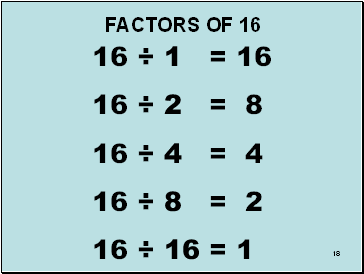18

16 ÷ 1 = 16

16 ÷ 2 = 8

16 ÷ 4 = 4

16 ÷ 8 = 2

16 ÷ 16 = 1

1

2

4

8

16

FACTORS OF 16

Slide 19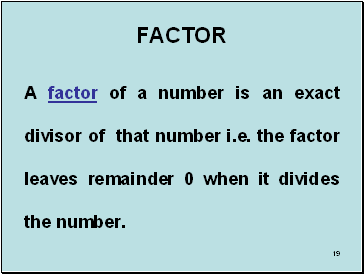19

## Factor

A factor of a number is an exact divisor of that number i.e. the factor leaves remainder 0 when it divides the number.

Slide 20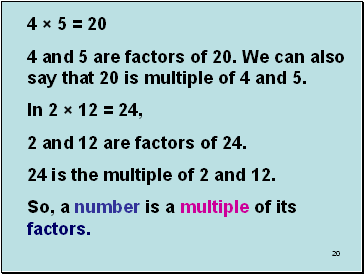20

4 × 5 = 20

4 and 5 are factors of 20. We can also say that 20 is multiple of 4 and 5.

In 2 × 12 = 24,

2 and 12 are factors of 24.

24 is the multiple of 2 and 12.

So, a number is a multiple of its factors.

Slide 21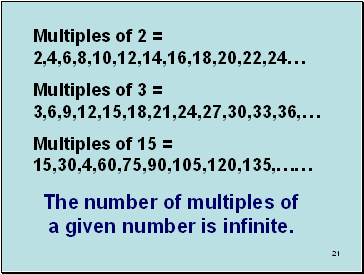21

Multiples of 2 = 2,4,6,8,10,12,14,16,18,20,22,24…

Multiples of 3 = 3,6,9,12,15,18,21,24,27,30,33,36,…

Multiples of 15 = 15,30,4,60,75,90,105,120,135,……

The number of multiples of a given number is infinite.

Slide 22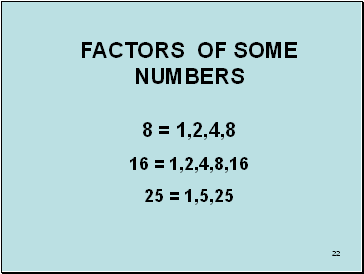22

## Factors of some numbers

8 = 1,2,4,8

16 = 1,2,4,8,16

25 = 1,5,25

Slide 23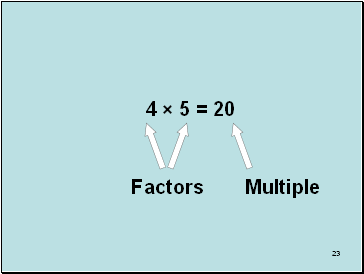23

4 × 5 = 20

Factors

Multiple

Slide 24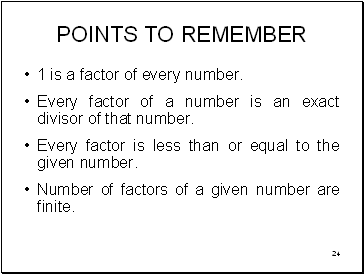24

## Points to remember

1 is a factor of every number.

Every factor of a number is an exact divisor of that number.

Every factor is less than or equal to the given number.

Number of factors of a given number are finite.

Slide 25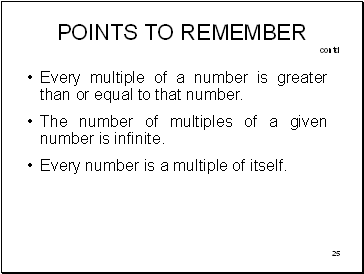25

Every multiple of a number is greater than or equal to that number.

The number of multiples of a given number is infinite.

Every number is a multiple of itself.

POINTS TO REMEMBER contd

Slide 26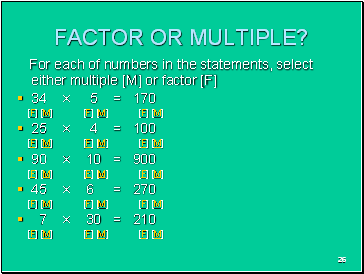26

## Factor or multiple?

For each of numbers in the statements, select either multiple [M] or factor [F]

34 × 5 = 170

[F] [M] [F] [M] [F] [M]

25 × 4 = 100

[F] [M] [F] [M] [F] [M]

90 × 10 = 900

[F] [M] [F] [M] [F] [M]

45 × 6 = 270

[F] [M] [F] [M] [F] [M]

7 × 30 = 210

[F] [M] [F] [M] [F] [M]

Slide 27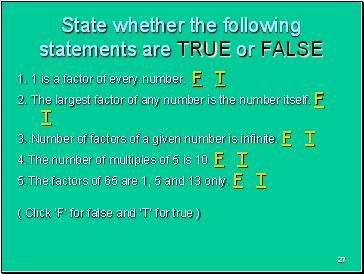27

State whether the following statements are TRUE or FALSE

1. 1 is a factor of every number. F T

Go to page:
1  2  3